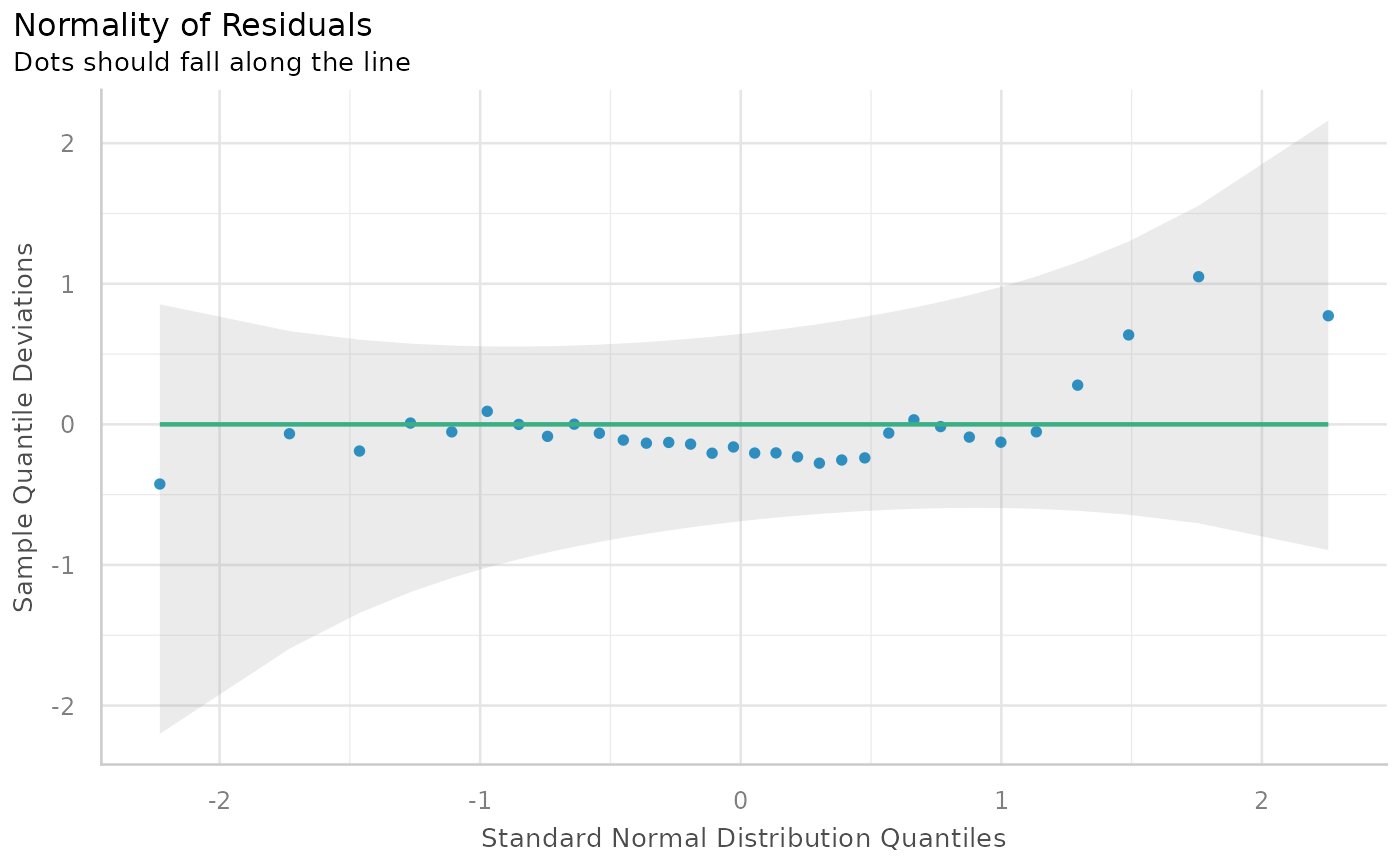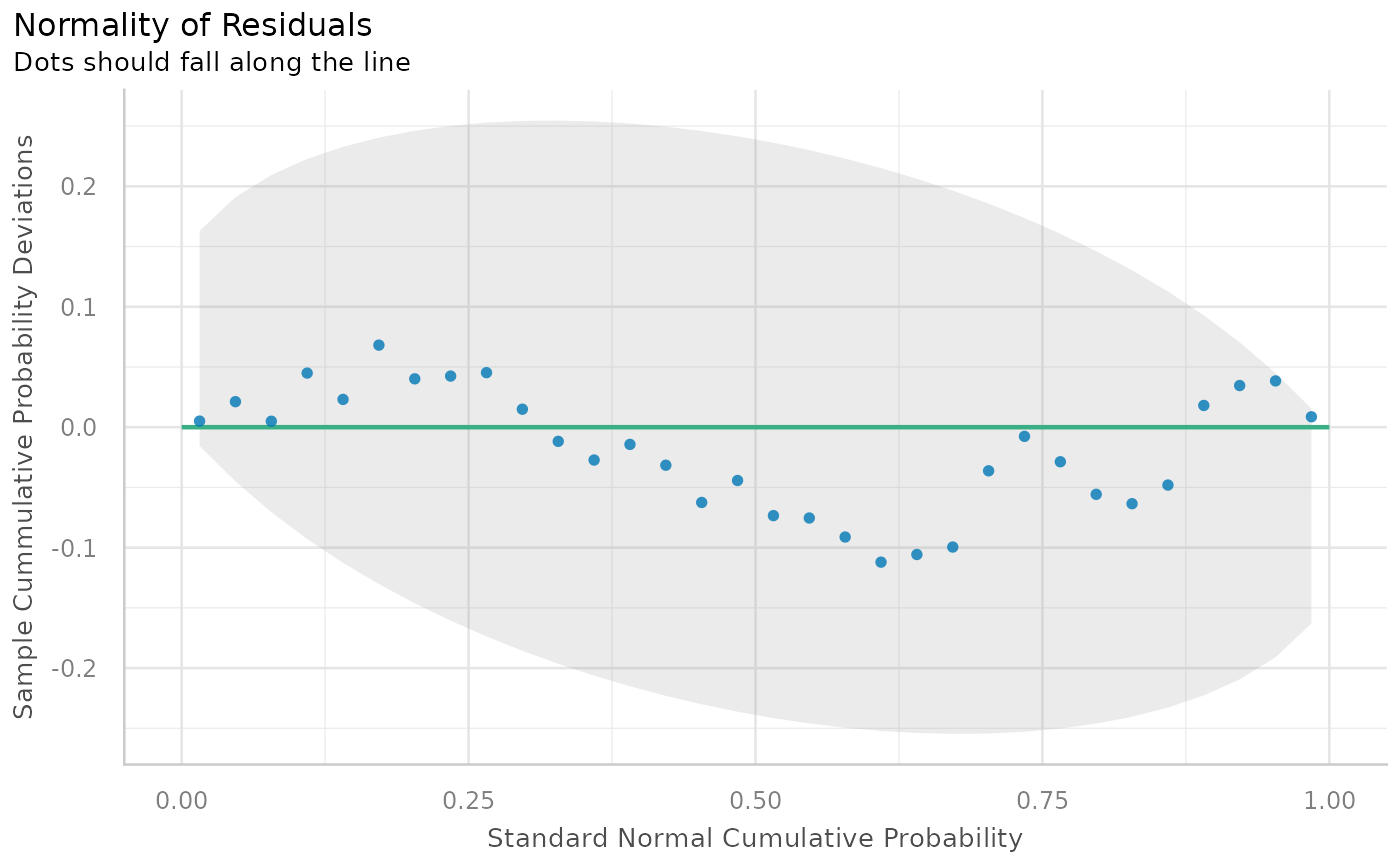Check model for (non-)normality of residuals.

## Usage

check_normality(x, ...)

# S3 method for merMod
check_normality(x, effects = c("fixed", "random"), ...)

## Arguments

x

A model object.

...

Currently not used.

effects

Should normality for residuals ("fixed") or random effects ("random") be tested? Only applies to mixed-effects models. May be abbreviated.

## Value

The p-value of the test statistics. A p-value < 0.05 indicates a significant deviation from normal distribution.

## Details

check_normality() calls stats::shapiro.test and checks the standardized residuals (or studentized residuals for mixed models) for normal distribution. Note that this formal test almost always yields significant results for the distribution of residuals and visual inspection (e.g. Q-Q plots) are preferable. For generalized linear models, no formal statistical test is carried out. Rather, there's only a plot() method for GLMs. This plot shows a half-normal Q-Q plot of the absolute value of the standardized deviance residuals is shown (in line with changes in plot.lm() for R 4.3+).

## Note

For mixed-effects models, studentized residuals, and not standardized residuals, are used for the test. There is also a plot()-method implemented in the see-package.

## Examples

m <<- lm(mpg ~ wt + cyl + gear + disp, data = mtcars)
check_normality(m)
#> OK: residuals appear as normally distributed (p = 0.230).
#>

# plot results
x <- check_normality(m)
plot(x)# \donttest{
# QQ-plot
plot(check_normality(m), type = "qq")# PP-plot
plot(check_normality(m), type = "pp")# }